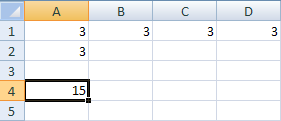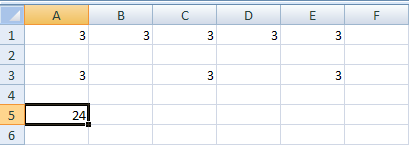# The Excel SUM Function

In earlier parts of this course, you used addition formula quite a lot. You saw that the basic way to add things up was by doing this:

=A1 + B1 + C1

You've also used the in-built SUM function:

=SUM(A1:C1)

Whichever of these two you used, the answer was the same - Excel will add up whatever numbers you have in the cells A1, B1, and C1. The two methods above are adding up consecutive cells. But what if you want to add up the following, non-consecutive cells: A1, B1, C1, D9?

Well, you can combine the two methods. So you can do it like this:

= Sum(A1:C1) + D9

or you can do it like this:

= Sum(A1:C1, D9)

For the first method, just type a plus sign after your SUM function, followed by the cell you want to include:

= Sum(A1:C1) + D9

You can include as many other cells as you like:

= Sum(A1:C1) + D9 + E12 + G25

You can even use another SUM function:

= Sum(A1:C1) + SUM(G1:H1)

The second method to add up non-consecutive cells starts in the same way: use a SUM function, and separate your consecutive cells with a colon:

= Sum(A1:C1)

To include the non consecutive cells, type a comma, followed by the cell you want to include:

= Sum(A1:C1, D9)

You can include other cells, as well:

= Sum(A1:C1, D9, E12, G25)

The thing to note is that all the cells are between the round brackets of the SUM function. Excel knows that SUM means to add up, so it sees each cell reference separated by commas, and then includes them in the addition.

To give you some practice, try this exercise.

Exercise

Create a simple spreadsheet with the number 3 in cells A1, B1, C1 and D1. Enter another number 3 in cell A2. Use one of the non consecutive addition formulas above to add up the values in all five cells. Your spreadsheet will then look like this, once you have the correct formula:In the picture above, cell A4 displays the correct answer.

### Selecting Non Consecutive Cells

Another way to select non-consecutive cells for your SUM functions is by holding down the CTRL key on your keyboard, and then left click in the cell you want to add. Try this:

• Click inside a different cell in your spreadsheet (B4, for example). Then click inside the formula bar at the top
• Now type the following into the formula bar (Don't forget to add the colon at the end):

=SUM(A1:

• The cell A1 will be highlighted on the spreadsheet. It will have sizing handles, so that you can stretch the selection
• Hold your left mouse button over the bottom right blue square, and drag to cell D1. You spreadsheet should look like this:Excel will add the cells to your formula. But it will also add a colon after D2. We don't want this, because a colon means "add up a range of cells". So delete the colon and type a comma instead.

Now that you have the cells A1 to D1 selected, hold down the left CTRL key on your keyboard. Keep it held down, and click inside cell A2 with your left mouse button:The cell A2 is highlighted, in the image above. Excel will add this to your formula.. To finish off, add the right bracket ). Then press the enter key on your keyboard.
Using this method, you can add as many individual cells as you want for your formula.

Exercise

On a new sheet, enter the number 3 in the following cells: A1, B1, C1, D1, E1. Then type a 3 in the cells A3, C3 and E3. Using non-consecutive addition, display your answer in cell A5. The finished spreadsheet will then look like ours below:The answer to the addition, 24, is displayed in A5. Only one Sum function was used here, with the other cells separated by commas.

Adding up shouldn't cause you too many problems. The tricky part is selecting all the cells that you want to include. In the next part, we'll at multiplication.

<--Back to the Excel Contents Page